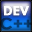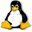Home Latest News Archive Tutorials C++ 2 3 4 Win32 Java OpenGL GLUT OpenGL ES 2 3 4 DirectX VB.NET HTML Photoshop 3dsmax Forums Projects Modelling Links About Us Search Recent Tutorials C / C++ Win32 OpenGL OpenGL ES GLUT## Function Templates

Tutorials > C++ > Function Templates

### Introduction

Function templates allow you to create a function that can accept or return more than one data type. This is useful if you have an operation that will work with a number of data types but you do not want to have a number of different functions with the same body but different parameters and return value.

Contents of main.cpp :

```#include <iostream>
#include <stdlib.h>

using namespace std;
```

To create a function template, you need to add the following line above your function :

template<class T>

where T can be any identifier. Wherever you now see T, you can imagine that T can be substituted with any data type.

As you see below, you can now use T wherever you would normally use your data type. This function allows any 2 variables of the same data type to be subtracted from each other and returned as a result of the same data type.

```template<class T>
T difference(T v1, T v2)
{
return v1 - v2;
}
```

We can see how different data types can be used in the code below. Our examples show how both integers and floats can be passed on to this function.

```int main()
{
int idif = difference(6, 9);
cout << idif << endl;

float fdif = difference(5.5f, 3.0f);
cout << fdif << endl;

system("pause");

return 0;
}```

Congratulations. You should now know how to create basic function templates. We will return to templates in a later tutorial and explain them more in detail. Function templates allow you to save a great amount of time as you can create just one function that will accept a number of data types. This also makes your code more readable and easier to understand.

Please let me know of any comments you may have : Contact Me

 Source Files :< Tutorial 44 - Namespaces Tutorial 46 - Classes >